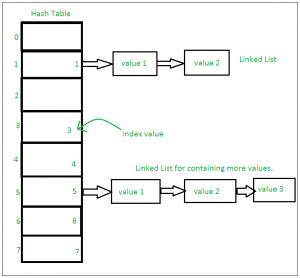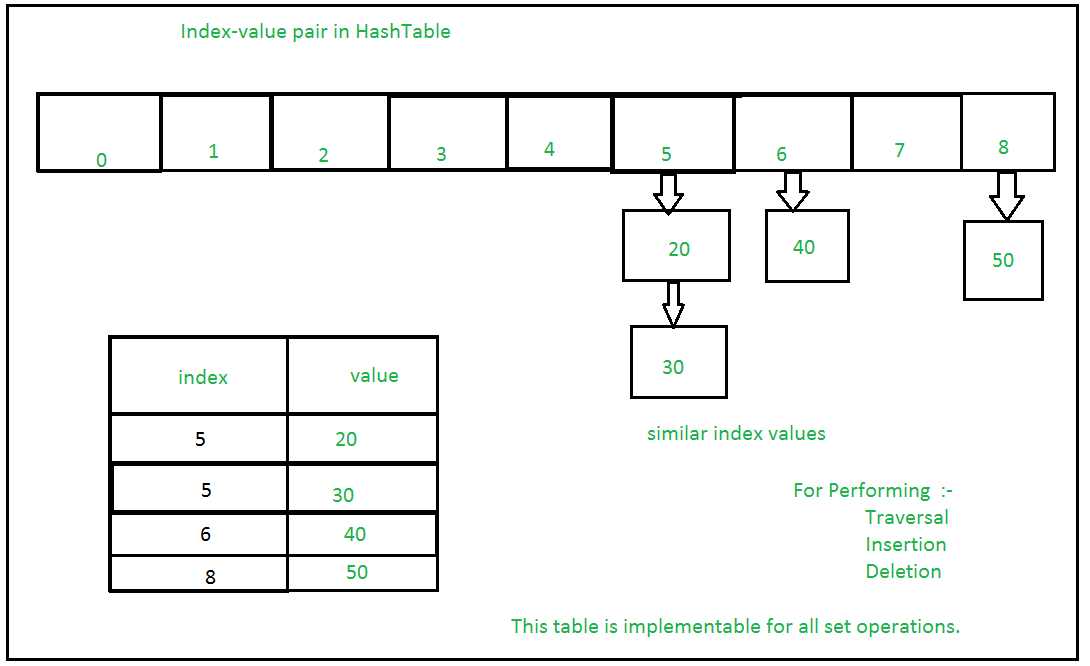1587358080

Learn Python Programming - Sets in Python

Sets are a key way to store data in Python. In this tutorial, we show you how to create sets, how to add and remove elements from a set, and how to take the union and intersection of two sets. We will talk about lists, dictionaries and tuples in separate videos.

A Set is an unordered collection data type that is iterable, mutable and has no duplicate elements. Python’s set class represents the mathematical notion of a set. The major advantage of using a set, as opposed to a list, is that it has a highly optimized method for checking whether a specific element is contained in the set. This is based on a data structure known as a hash table. Since sets are unordered, we cannot access items using indexes like we do in lists.

# Python program to
# demonstrate sets

# Same as {"a", "b", "c"}
myset = set(["a", "b", "c"])
print(myset)

# Adding element to the set
print(myset)

Output:

{'c', 'b', 'a'}
{'d', 'c', 'b', 'a'}

Frozen Sets

Frozen sets in Python are immutable objects that only support methods and operators that produce a result without affecting the frozen set or sets to which they are applied. While elements of a set can be modified at any time, elements of the frozen set remain the same after creation.
If no parameters are passed, it returns an empty frozenset.

# Python program to demonstrate differences
# between normal and frozen set

# Same as {"a", "b","c"}
normal_set = set(["a", "b","c"])

print("Normal Set")
print(normal_set)

# A frozen set
frozen_set = frozenset(["e", "f", "g"])

print("\nFrozen Set")
print(frozen_set)

# Uncommenting below line would cause error as
# we are trying to add element to a frozen set

Output:

Normal Set
set(['a', 'c', 'b'])

Frozen Set
frozenset(['e', 'g', 'f'])

Internal working of Set

This is based on a data structure known as a hash table.
If Multiple values are present at the same index position, then the value is appended to that index position, to form a Linked List. In, Python Sets are implemented using dictionary with dummy variables, where key beings the members set with greater optimizations to the time complexity.

Set Implementation:-Sets with Numerous operations on a single HashTable:-Methods for Sets

Insertion in set is done through set.add() function, where an appropriate record value is created to store in the hash table. Same as checking for an item, i.e., O(1) on average. However, in worst case it can become O(n).

# A Python program to
# in a set

# Creating a Set
people = {"Jay", "Idrish", "Archi"}

print("People:", end = " ")
print(people)

# in the set

# set using iterator
for i in range(1, 6):

print("\nSet after adding element:", end = " ")
print(people)

Output:

People: {'Idrish', 'Archi', 'Jay'}

Set after adding element: {1, 2, 3, 4, 5, 'Idrish', 'Archi', 'Jay', 'Daxit'}

Union

Two sets can be merged using union() function or | operator. Both Hash Table values are accessed and traversed with merge operation perform on them to combine the elements, at the same time duplicates are removed. Time Complexity of this is O(len(s1) + len(s2)) where s1 and s2 are two sets whose union needs to be done.

# Python Program to
# demonstrate union of
# two sets

people = {"Jay", "Idrish", "Archil"}
vampires = {"Karan", "Arjun"}
dracula = {"Deepanshu", "Raju"}

# Union using union()
# function
population = people.union(vampires)

print("Union using union() function")
print(population)

# Union using "|"
# operator
population = people|dracula

print("\nUnion using '|' operator")
print(population)

Output:

Union using union() function
{'Karan', 'Idrish', 'Jay', 'Arjun', 'Archil'}

Union using '|' operator
{'Deepanshu', 'Idrish', 'Jay', 'Raju', 'Archil'}

Intersection

This can be done through intersection() or & operator. Common Elements are selected. They are similar to iteration over the Hash lists and combining the same values on both the Table. Time Complexity of this is O(min(len(s1), len(s2)) where s1 and s2 are two sets whose union needs to be done.

# Python program to
# demonstrate intersection
# of two sets

set1 = set()
set2 = set()

for i in range(5):

for i in range(3,9):

# Intersection using
# intersection() function
set3 = set1.intersection(set2)

print("Intersection using intersection() function")
print(set3)

# Intersection using
# "&" operator
set3 = set1 & set2

print("\nIntersection using '&' operator")
print(set3)

Output:

Intersection using intersection() function
{3, 4}

Intersection using '&' operator
{3, 4}

Difference

To find difference in between sets. Similar to find difference in linked list. This is done through difference() or – operator. Time complexity of finding difference s1 – s2 is O(len(s1))

# Python program to
# demonstrate difference
# of two sets

set1 = set()
set2 = set()

for i in range(5):

for i in range(3,9):

# Difference of two sets
# using difference() function
set3 = set1.difference(set2)

print(" Difference of two sets using difference() function")
print(set3)

# Difference of two sets
# using '-' operator
set3 = set1 - set2

print("\nDifference of two sets using '-' operator")
print(set3)

Output:

Difference of two sets using difference() function
{0, 1, 2}

Difference of two sets using '-' operator
{0, 1, 2}

Clearing sets

Clear() method empties the whole set.

# Python program to
# demonstrate clearing
# of set

set1 = {1,2,3,4,5,6}

print("Initial set")
print(set1)

# This method will remove
# all the elements of the set
set1.clear()

print("\nSet after using clear() function")
print(set1)

Output:

Initial set
{1, 2, 3, 4, 5, 6}

Set after using clear() function
set()

However, there are two major pitfalls in Python sets:

1. The set doesn’t maintain elements in any particular order.
2. Only instances of immutable types can be added to a Python set.

Time complexity of SetsOperators for Sets

Sets and frozen sets support the following operators:Code Snippet to illustrate all Set operations in Python

# Python program to demonstrate working# of
# Set in Python

# Creating two sets
set1 = set()
set2 = set()

for i in range(1, 6):

for i in range(3, 8):

print("Set1 = ", set1)
print("Set2 = ", set2)
print("\n")

# Union of set1 and set2
set3 = set1 | set2# set1.union(set2)
print("Union of Set1 & Set2: Set3 = ", set3)

# Intersection of set1 and set2
set4 = set1 & set2# set1.intersection(set2)
print("Intersection of Set1 & Set2: Set4 = ", set4)
print("\n")

# Checking relation between set3 and set4
if set3 > set4: # set3.issuperset(set4)
print("Set3 is superset of Set4")
elif set3 < set4: # set3.issubset(set4)
print("Set3 is subset of Set4")
else : # set3 == set4
print("Set3 is same as Set4")

# displaying relation between set4 and set3
if set4 < set3: # set4.issubset(set3)
print("Set4 is subset of Set3")
print("\n")

# difference between set3 and set4
set5 = set3 - set4
print("Elements in Set3 and not in Set4: Set5 = ", set5)
print("\n")

# checkv if set4 and set5 are disjoint sets
if set4.isdisjoint(set5):
print("Set4 and Set5 have nothing in common\n")

# Removing all the values of set5
set5.clear()

print("After applying clear on sets Set5: ")
print("Set5 = ", set5)

Output:

('Set1 = ', set([1, 2, 3, 4, 5]))
('Set2 = ', set([3, 4, 5, 6, 7]))

('Union of Set1 & Set2: Set3 = ', set([1, 2, 3, 4, 5, 6, 7]))
('Intersection of Set1 & Set2: Set4 = ', set([3, 4, 5]))

Set3 is superset of Set4
Set4 is subset of Set3

('Elements in Set3 and not in Set4: Set5 = ', set([1, 2, 6, 7]))

Set4 and Set5 have nothing in common

After applying clear on sets Set5:
('Set5 = ', set([]))

#python #web-development #machine-learning

Buddha Community1619518440

top 30 Python Tips and Tricks for Beginners

Welcome to my Blog , In this article, you are going to learn the top 10 python tips and tricks.

8) Check The Memory Usage Of An Object.

#python #python hacks tricks #python learning tips #python programming tricks #python tips #python tips and tricks #python tips and tricks advanced #python tips and tricks for beginners #python tips tricks and techniques #python tutorial #tips and tricks in python #tips to learn python #top 30 python tips and tricks for beginners1625843760

Python Packages in SQL Server – Get Started with SQL Server Machine Learning Services

Introduction

When installing Machine Learning Services in SQL Server by default few Python Packages are installed. In this article, we will have a look on how to get those installed python package information.

Python Packages

When we choose Python as Machine Learning Service during installation, the following packages are installed in SQL Server,

• revoscalepy – This Microsoft Python package is used for remote compute contexts, streaming, parallel execution of rx functions for data import and transformation, modeling, visualization, and analysis.
• microsoftml – This is another Microsoft Python package which adds machine learning algorithms in Python.
• Anaconda 4.2 – Anaconda is an opensource Python package

#machine learning #sql server #executing python in sql server #machine learning using python #machine learning with sql server #ml in sql server using python #python in sql server ml #python packages #python packages for machine learning services #sql server machine learning services1602154800

Learning Python: The Prompt, Then Read Template

In my last article I discussed how to use the Input-Process-Output template as a general guide to how programs should be structured in Python. To review, most Python programs will consist of three steps — getting input into the program, processing the input in some way, and outputting the results of the processing.

In this article, I’m going to focus on one part of that step — getting input into a program — by prompting the user to enter some data and then reading the data into the program. This is a mostly straightforward process except for some data conversions that have to occur when you are inputting numbers.

Defining a Prompt

Let’s start by defining the word prompt. A prompt is a message to the user of your program telling them what they are supposed to be entering into the program. A prompt needs to be descriptive but doesn’t have to be overly detailed.

For example, if your program needs the user to enter their name, you can use a prompt like this:

However, you may need to be more specific if you want the user to enter their first name and their last name separately. The more appropriate prompt in this case might really be two prompts:

#learn-to-code #learn-python-programming #python #learning-python #python-programming1619510796

Lambda, Map, Filter functions in python

Welcome to my Blog, In this article, we will learn python lambda function, Map function, and filter function.

Lambda function in python: Lambda is a one line anonymous function and lambda takes any number of arguments but can only have one expression and python lambda syntax is

Syntax: x = lambda arguments : expression

Now i will show you some python lambda function examples:

#python #anonymous function python #filter function in python #lambda #lambda python 3 #map python #python filter #python filter lambda #python lambda #python lambda examples #python map1574340826

Learn Python Programming

Description
Learn Python Programming

Learn Python Programming and increase your python programming skills with Coder Kovid.

Python is the highest growing programming language in this era. You can use Python to do everything like, web development, software development, cognitive development, machine learning, artificial intelligence, etc. You should learn python programming and increase your skills of programming.

In this course of learn python programming you don’t need any prior programming knowledge. Every beginner can start with.

Basic knowledge
No prior knowledge needed to learn this course
What will you learn
Write Basic Syntax of Python Programming
Create Basic Real World Application
Program in a fluent manner
Get Familiar in Programming Environment

#Learn Python Programming # Learn Python #Python Programming # Programming